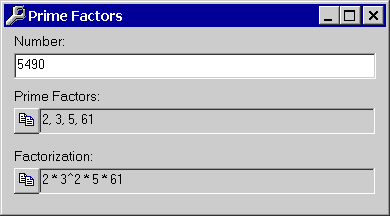Prime Factorization Calculator

The image shown is the prime factorization calculator, which allows the user to determine if a number is prime or composite. The calculator also provides the prime factors, and the prime factorization (prime factors with powers)Math tools included in the Mega Toolbox are:
• Least Common Multiple and Greatest Common Factor calculator
• Base converter
• Happy Number Calculator
• Polar and Rectangular Calculator
• Prime Factorization Calculator